# Analog Circuits - Online Test

Q1.
The approximate transfer characteristic for the circuit shown below with an ideal operational amplifier and diode will be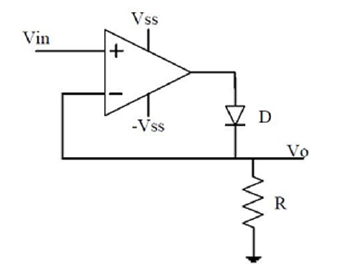Explaination / Solution:
No Explaination.

Q2. Given that the op-amp is ideal, the output voltage V0 isExplaination / Solution:
No Explaination.

Q3. The nature of feedback in the op-amp circuit shown is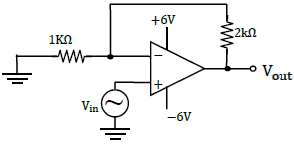Explaination / Solution:
No Explaination.

Q4. The following circuit has R = 10kΩ, C = 10μF . The input voltage is a sinusoid at 50Hz with an rms value of 10V. Under ideal conditions, the current is from the source is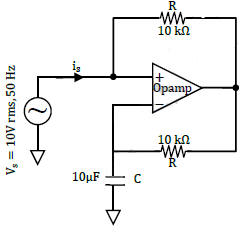Explaination / Solution:
No Explaination.

Q5. In a transconductance amplifier, it is desirable to have
Explaination / Solution:

In the transconductance amplifier it is desirable to have large input resistance and large output resistance.

Q6. For the Op-Amp circuit shown in the figure, V is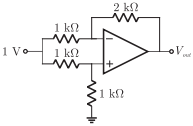Explaination / Solution:
No Explaination.

Q7. In the Op-Amp circuit shown, assume that the diode current follows the equation I = Ise P(V VT). For V= V, V = V, and for V= V, V = V. The relationship between V and V is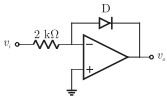Explaination / Solution:

Here the inverting terminal is at virtual ground and the current in resistor and diode current is equal i.e.Q8. Consider the Op-Amp circuit shown in the figure.The transfer function V(s) Vi(s)
Explaination / Solution:Q9. Consider the Op-Amp circuit shown in the figure.If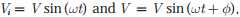then the minimum and maximum values of ϕ (in radians) are respectively
Explaination / Solution:Q10. In the Digital-to-Analog converter circuit shown in the figure below, VR = 1V and R = 1kΩ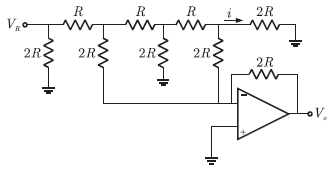The voltage V  is
Explaination / Solution:
No Explaination.